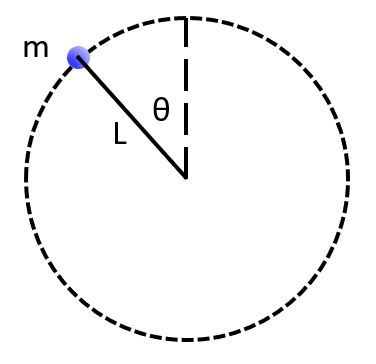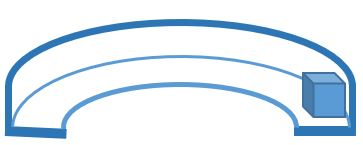PH 212

Uniform circular motion applications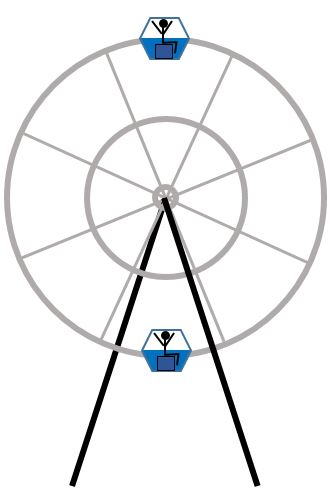A person rides on a Ferris wheel of radius r at constant speed v. (Image is exaggerated. Assume the person is on the outer rim of the wheel.)

How does the normal force exerted on the rider at the top compare to the normal force on the rider at the bottom?

Consider a car of mass m, going around a curve of radius r at a constant speed v. What is its acceleration?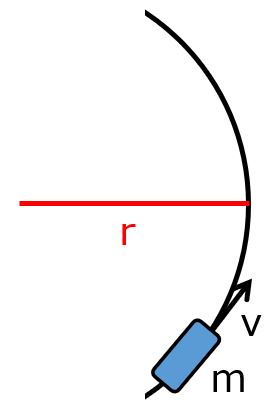What assumptions can you make about the system above?

X. There is no air resistance.
Y. There is no friction.

A. Only X is true.

B. Only Y is true.

C. Both X and Y are true.

D. Neither X nor Y is true.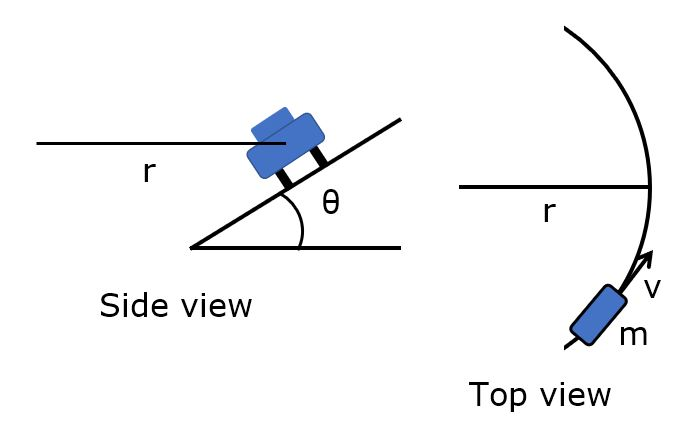Side view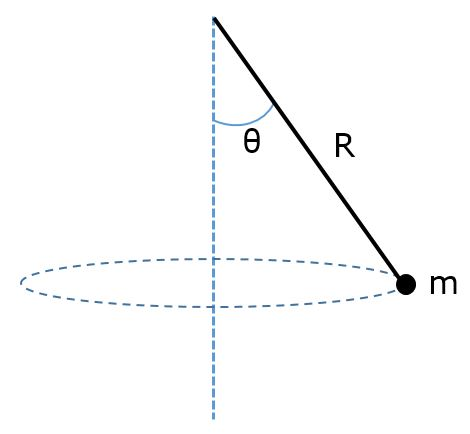A ball of mass m is tied to the end of a string of length L and swung in a vertical clockwise circle. The center of the circle is a distance h above the floor. The ball is swung at the minimum speed necessary to make it over the top without the string going slack. If the string is released at the moment the ball is at the top of the circle, how far to the right does the ball hit the floor?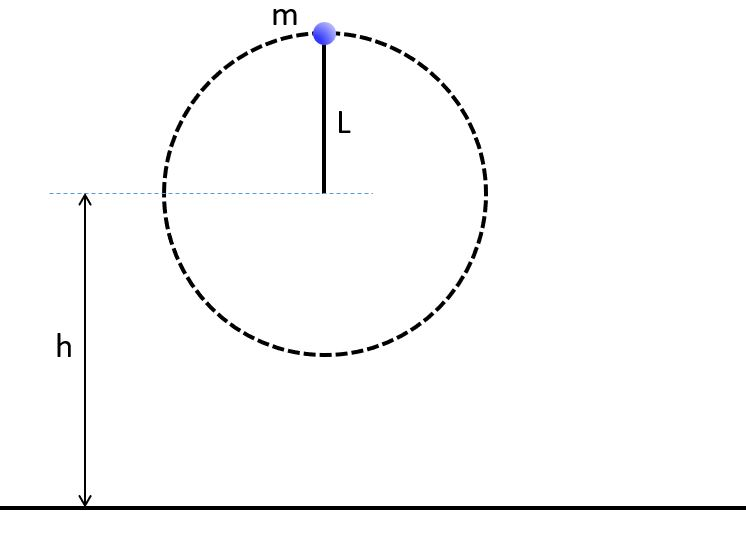A ball of mass m is tied to the end of a string of length L and swung in a vertical clockwise circle. At the instant the ball is at angle t as shown, there is a tension T in the string. What is the magnitude and direction of the acceleration of the ball?